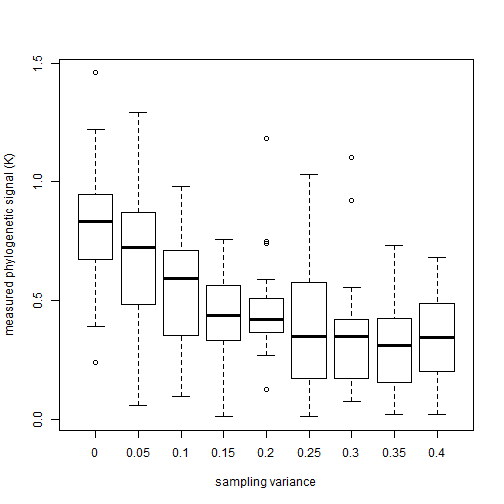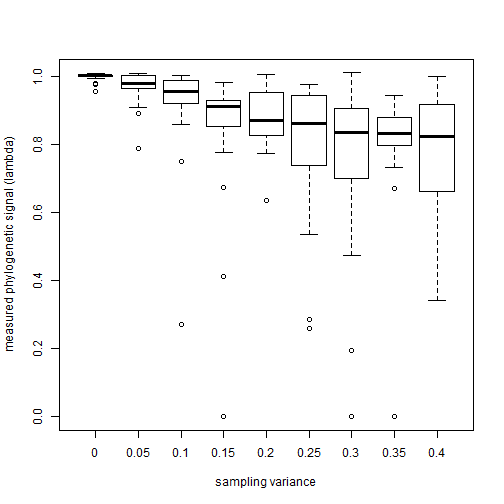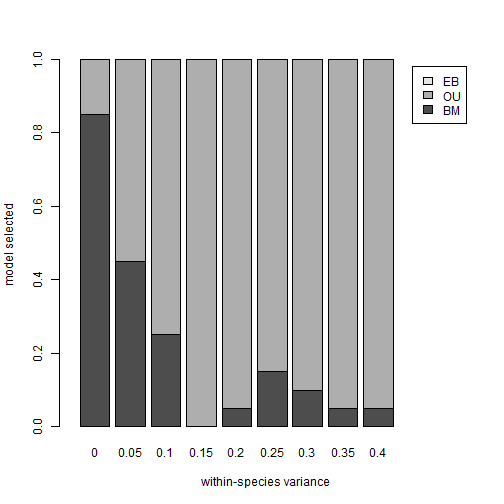## Solution to Challenge Problem 3: Fitting models of continuous character evolution

In the challenge problem I asked you to first download two files for cyprinid fishes (Cyprinidae-data.csv, and Cyprinidae-tree.tre).Then you were asked to estimate phylogenetic signal by different metrics (K, λ), and to fit BM, OU, & EB models to the dataset. The second column of the data matrix contained the standard errors of each species mean, so you were asked to read the relevant documentation files of `phylosig` and `fitContinuous` to figure out how that can be taken into account. Finally, you were asked to assess which model fit best.

First, phylogenetic signal:

``````## packages
library(phytools)
library(geiger)

## remove taxa from the tree not in the dataset & vice versa
obj<-name.check(cyprinid.tree,X)
obj
``````
``````## \$tree_not_data
##  "CcomA"    "Danio_Li" "PeryA"
##
## \$data_not_tree
## character(0)
``````
``````cyprinid.tree<-drop.tip(cyprinid.tree,obj\$tree_not_data)

## extract the head lengths and SEs
hl<-setNames(X[,1],rownames(X))
se<-setNames(X[,2],rownames(X))

## estimate phylogenetic signal
phylosig(cyprinid.tree,hl,se=se)
``````
``````## \$K
##  1.269532
##
## \$sig2
##  11.92121
##
## \$logL
##  -77.95265
``````
``````phylosig(cyprinid.tree,hl,method="lambda",se=se)
``````
``````## \$lambda
##  1.018471
##
## \$sig2
##  11.98363
##
## \$logL
##  -77.9213
##
## \$convergence
##  0
##
## \$message
##  "CONVERGENCE: REL_REDUCTION_OF_F <= FACTR*EPSMCH"
``````

Next model fitting using `fitContinuous`:

``````## fit models
fitBM<-fitContinuous(cyprinid.tree,hl,SE=se)
fitOU<-fitContinuous(cyprinid.tree,hl,SE=se,model="OU")
fitEB<-fitContinuous(cyprinid.tree,hl,SE=se,model="EB")

## examine models
fitBM
``````
``````## GEIGER-fitted comparative model of continuous data
##  fitted 'BM' model parameters:
##  sigsq = 11.580627
##  z0 = 13.401065
##
##  model summary:
##  log-likelihood = -77.952650
##  AIC = 159.905301
##  AICc = 160.280301
##  free parameters = 2
##
## Convergence diagnostics:
##  optimization iterations = 100
##  failed iterations = 0
##  frequency of best fit = 1.00
##
##  object summary:
##  'lik' -- likelihood function
##  'bnd' -- bounds for likelihood search
##  'res' -- optimization iteration summary
##  'opt' -- maximum likelihood parameter estimates
``````
``````fitOU
``````
``````## GEIGER-fitted comparative model of continuous data
##  fitted 'OU' model parameters:
##  alpha = 0.000000
##  sigsq = 11.580628
##  z0 = 13.401065
##
##  model summary:
##  log-likelihood = -77.952650
##  AIC = 161.905301
##  AICc = 162.679494
##  free parameters = 3
##
## Convergence diagnostics:
##  optimization iterations = 100
##  failed iterations = 0
##  frequency of best fit = 0.63
##
##  object summary:
##  'lik' -- likelihood function
##  'bnd' -- bounds for likelihood search
##  'res' -- optimization iteration summary
##  'opt' -- maximum likelihood parameter estimates
``````
``````fitEB
``````
``````## GEIGER-fitted comparative model of continuous data
##  fitted 'EB' model parameters:
##  a = -1.245004
##  sigsq = 27.407037
##  z0 = 13.472784
##
##  model summary:
##  log-likelihood = -77.812985
##  AIC = 161.625970
##  AICc = 162.400164
##  free parameters = 3
##
## Convergence diagnostics:
##  optimization iterations = 100
##  failed iterations = 0
##  frequency of best fit = 0.20
##
##  object summary:
##  'lik' -- likelihood function
##  'bnd' -- bounds for likelihood search
##  'res' -- optimization iteration summary
##  'opt' -- maximum likelihood parameter estimates
``````

Finally, relative model fit:

``````## compare AICs and compute AIC weights
aic.vals<-setNames(c(fitBM\$opt\$aicc,fitOU\$opt\$aicc,fitEB\$opt\$aicc),
c("BM","OU","EB"))
aic.vals
``````
``````##       BM       OU       EB
## 160.2803 162.6795 162.4002
``````
``````aic.w<-function(aic){
d.aic<-aic-min(aic)
exp(-1/2*d.aic)/sum(exp(-1/2*d.aic))
}
aic.w(aic.vals)
``````
``````##        BM        OU        EB
## 0.6068715 0.1828599 0.2102686
``````

This shows that the weight of evidence supports the BM model over the alternatives that we have considered.

We can also explore the effect of ignoring error in the estimation of species means on phylogenetic signal & model selection for comparative analysis.

First, let's consider how to simulate data with measurement error in the estimation of species means. To do this, let's simulate species means and then simulate error in the estimation of those means by drawing it randomly.

``````N<-50
nsim<-20
trees<-pbtree(n=N,scale=1,nsim=nsim)
trees
``````
``````## 20 phylogenetic trees
``````
``````x<-lapply(trees,fastBM)
fn<-function(trees,x,sampling.var){
## variances for random error:
foo<-function() rep(sampling.var,N)/sample(1:5,size=N,replace=TRUE)
v<-replicate(nsim,foo(),simplify=FALSE)
foo<-function(x,v) mapply(sampleFrom,xbar=x,xvar=v)
mapply(foo,x=x,v=v,SIMPLIFY=FALSE)
}
``````

Now let's compute K & λ for various levels of sampling error:

``````ve<-seq(0,0.4,by=0.05)
K<-lambda<-matrix(NA,nsim,length(ve),dimnames=list(NULL,ve))
xe<-list()
for(i in 1:length(ve)){
xe[[i]]<-fn(trees,x,ve[i])
K[,i]<-mapply(phylosig,tree=trees,x=xe[[i]])
foo<-function(tree,x) phylosig(tree,x,method="lambda")\$lambda
lambda[,i]<-mapply(foo,tree=trees,x=xe[[i]])
}
colMeans(K)
``````
``````##         0      0.05       0.1      0.15       0.2      0.25       0.3
## 0.8365042 0.6790406 0.5472466 0.4367745 0.4711542 0.3912281 0.3676326
##      0.35       0.4
## 0.3195309 0.3547007
``````
``````colMeans(lambda)
``````
``````##         0      0.05       0.1      0.15       0.2      0.25       0.3
## 0.9974198 0.9666582 0.9107633 0.8261313 0.8766996 0.7969854 0.7463530
##      0.35       0.4
## 0.7933736 0.7729163
``````
``````boxplot(K,xlab="sampling variance",ylab="measured phylogenetic signal (K)")
````````````boxplot(lambda,xlab="sampling variance",ylab="measured phylogenetic signal (lambda)")
``````So we can see that ignored sampling error tends to systematically bias downwardly the measurement of phylogenetic signal by both of these metrics.

Next, let's explore the effects of ignored sampling error on model selection for alternative models using `fitContinuous`:

``````best.model<-matrix(NA,nsim,length(ve),dimnames=list(NULL,ve))
for(i in 1:length(ve)){
print(ve[i])
fitBM<-mapply(fitContinuous,phy=trees,dat=xe[[i]],SIMPLIFY=FALSE)
fitOU<-mapply(fitContinuous,phy=trees,dat=xe[[i]],
MoreArgs=list(model="OU"),SIMPLIFY=FALSE)
fitEB<-mapply(fitContinuous,phy=trees,dat=xe[[i]],
MoreArgs=list(model="EB"),SIMPLIFY=FALSE)
foo<-function(fit1,fit2,fit3){
aic<-setNames(c(fit1\$opt\$aicc,fit2\$opt\$aicc,fit3\$opt\$aicc),
c("BM","OU","EB"))
ii<-which(aic==min(aic))
names(aic)[ii]
}
best.model[,i]<-mapply(foo,fit1=fitBM,fit2=fitOU,fit3=fitEB)
}
``````
``````##  0
``````
``````## Warning in (function (phy, dat, SE = 0, model = c("BM", "OU", "EB", "trend", : Parameter estimates appear at bounds:
##  alpha
``````
``````## Warning in (function (phy, dat, SE = 0, model = c("BM", "OU", "EB", "trend", : Parameter estimates appear at bounds:
##  a

## Warning in (function (phy, dat, SE = 0, model = c("BM", "OU", "EB", "trend", : Parameter estimates appear at bounds:
##  a

## Warning in (function (phy, dat, SE = 0, model = c("BM", "OU", "EB", "trend", : Parameter estimates appear at bounds:
##  a
``````
``````##  0.05
``````
``````## Warning in (function (phy, dat, SE = 0, model = c("BM", "OU", "EB", "trend", : Parameter estimates appear at bounds:
##  alpha
``````
``````## Warning in (function (phy, dat, SE = 0, model = c("BM", "OU", "EB", "trend", : Parameter estimates appear at bounds:
##  alpha

## Warning in (function (phy, dat, SE = 0, model = c("BM", "OU", "EB", "trend", : Parameter estimates appear at bounds:
##  alpha

## Warning in (function (phy, dat, SE = 0, model = c("BM", "OU", "EB", "trend", : Parameter estimates appear at bounds:
##  alpha
``````
``````## Warning in (function (phy, dat, SE = 0, model = c("BM", "OU", "EB", "trend", : Parameter estimates appear at bounds:
##  a

## Warning in (function (phy, dat, SE = 0, model = c("BM", "OU", "EB", "trend", : Parameter estimates appear at bounds:
##  a

## Warning in (function (phy, dat, SE = 0, model = c("BM", "OU", "EB", "trend", : Parameter estimates appear at bounds:
##  a

## Warning in (function (phy, dat, SE = 0, model = c("BM", "OU", "EB", "trend", : Parameter estimates appear at bounds:
##  a
``````
``````##  0.1
``````
``````## Warning in (function (phy, dat, SE = 0, model = c("BM", "OU", "EB", "trend", : Parameter estimates appear at bounds:
##  alpha
``````
``````## Warning in (function (phy, dat, SE = 0, model = c("BM", "OU", "EB", "trend", : Parameter estimates appear at bounds:
##  alpha

## Warning in (function (phy, dat, SE = 0, model = c("BM", "OU", "EB", "trend", : Parameter estimates appear at bounds:
##  alpha

## Warning in (function (phy, dat, SE = 0, model = c("BM", "OU", "EB", "trend", : Parameter estimates appear at bounds:
##  alpha

## Warning in (function (phy, dat, SE = 0, model = c("BM", "OU", "EB", "trend", : Parameter estimates appear at bounds:
##  alpha
``````
``````## Warning in (function (phy, dat, SE = 0, model = c("BM", "OU", "EB", "trend", : Parameter estimates appear at bounds:
##  a
``````
``````##  0.15
``````
``````## Warning in (function (phy, dat, SE = 0, model = c("BM", "OU", "EB", "trend", : Parameter estimates appear at bounds:
##  alpha
``````
``````## Warning in (function (phy, dat, SE = 0, model = c("BM", "OU", "EB", "trend", : Parameter estimates appear at bounds:
##  alpha

## Warning in (function (phy, dat, SE = 0, model = c("BM", "OU", "EB", "trend", : Parameter estimates appear at bounds:
##  alpha

## Warning in (function (phy, dat, SE = 0, model = c("BM", "OU", "EB", "trend", : Parameter estimates appear at bounds:
##  alpha

## Warning in (function (phy, dat, SE = 0, model = c("BM", "OU", "EB", "trend", : Parameter estimates appear at bounds:
##  alpha

## Warning in (function (phy, dat, SE = 0, model = c("BM", "OU", "EB", "trend", : Parameter estimates appear at bounds:
##  alpha

## Warning in (function (phy, dat, SE = 0, model = c("BM", "OU", "EB", "trend", : Parameter estimates appear at bounds:
##  alpha
``````
``````## Warning in (function (phy, dat, SE = 0, model = c("BM", "OU", "EB", "trend", : Parameter estimates appear at bounds:
##  a
``````
``````##  0.2
``````
``````## Warning in (function (phy, dat, SE = 0, model = c("BM", "OU", "EB", "trend", : Parameter estimates appear at bounds:
##  alpha
``````
``````## Warning in (function (phy, dat, SE = 0, model = c("BM", "OU", "EB", "trend", : Parameter estimates appear at bounds:
##  alpha

## Warning in (function (phy, dat, SE = 0, model = c("BM", "OU", "EB", "trend", : Parameter estimates appear at bounds:
##  alpha

## Warning in (function (phy, dat, SE = 0, model = c("BM", "OU", "EB", "trend", : Parameter estimates appear at bounds:
##  alpha
``````
``````## Warning in (function (phy, dat, SE = 0, model = c("BM", "OU", "EB", "trend", : Parameter estimates appear at bounds:
##  a

## Warning in (function (phy, dat, SE = 0, model = c("BM", "OU", "EB", "trend", : Parameter estimates appear at bounds:
##  a

## Warning in (function (phy, dat, SE = 0, model = c("BM", "OU", "EB", "trend", : Parameter estimates appear at bounds:
##  a
``````
``````##  0.25
``````
``````## Warning in (function (phy, dat, SE = 0, model = c("BM", "OU", "EB", "trend", : Parameter estimates appear at bounds:
##  alpha
``````
``````## Warning in (function (phy, dat, SE = 0, model = c("BM", "OU", "EB", "trend", : Parameter estimates appear at bounds:
##  alpha

## Warning in (function (phy, dat, SE = 0, model = c("BM", "OU", "EB", "trend", : Parameter estimates appear at bounds:
##  alpha

## Warning in (function (phy, dat, SE = 0, model = c("BM", "OU", "EB", "trend", : Parameter estimates appear at bounds:
##  alpha

## Warning in (function (phy, dat, SE = 0, model = c("BM", "OU", "EB", "trend", : Parameter estimates appear at bounds:
##  alpha

## Warning in (function (phy, dat, SE = 0, model = c("BM", "OU", "EB", "trend", : Parameter estimates appear at bounds:
##  alpha

## Warning in (function (phy, dat, SE = 0, model = c("BM", "OU", "EB", "trend", : Parameter estimates appear at bounds:
##  alpha

## Warning in (function (phy, dat, SE = 0, model = c("BM", "OU", "EB", "trend", : Parameter estimates appear at bounds:
##  alpha

## Warning in (function (phy, dat, SE = 0, model = c("BM", "OU", "EB", "trend", : Parameter estimates appear at bounds:
##  alpha

## Warning in (function (phy, dat, SE = 0, model = c("BM", "OU", "EB", "trend", : Parameter estimates appear at bounds:
##  alpha

## Warning in (function (phy, dat, SE = 0, model = c("BM", "OU", "EB", "trend", : Parameter estimates appear at bounds:
##  alpha
``````
``````## Warning in (function (phy, dat, SE = 0, model = c("BM", "OU", "EB", "trend", : Parameter estimates appear at bounds:
##  a

## Warning in (function (phy, dat, SE = 0, model = c("BM", "OU", "EB", "trend", : Parameter estimates appear at bounds:
##  a

## Warning in (function (phy, dat, SE = 0, model = c("BM", "OU", "EB", "trend", : Parameter estimates appear at bounds:
##  a
``````
``````##  0.3
``````
``````## Warning in (function (phy, dat, SE = 0, model = c("BM", "OU", "EB", "trend", : Parameter estimates appear at bounds:
##  alpha
``````
``````## Warning in (function (phy, dat, SE = 0, model = c("BM", "OU", "EB", "trend", : Parameter estimates appear at bounds:
##  alpha

## Warning in (function (phy, dat, SE = 0, model = c("BM", "OU", "EB", "trend", : Parameter estimates appear at bounds:
##  alpha

## Warning in (function (phy, dat, SE = 0, model = c("BM", "OU", "EB", "trend", : Parameter estimates appear at bounds:
##  alpha

## Warning in (function (phy, dat, SE = 0, model = c("BM", "OU", "EB", "trend", : Parameter estimates appear at bounds:
##  alpha

## Warning in (function (phy, dat, SE = 0, model = c("BM", "OU", "EB", "trend", : Parameter estimates appear at bounds:
##  alpha

## Warning in (function (phy, dat, SE = 0, model = c("BM", "OU", "EB", "trend", : Parameter estimates appear at bounds:
##  alpha

## Warning in (function (phy, dat, SE = 0, model = c("BM", "OU", "EB", "trend", : Parameter estimates appear at bounds:
##  alpha

## Warning in (function (phy, dat, SE = 0, model = c("BM", "OU", "EB", "trend", : Parameter estimates appear at bounds:
##  alpha

## Warning in (function (phy, dat, SE = 0, model = c("BM", "OU", "EB", "trend", : Parameter estimates appear at bounds:
##  alpha

## Warning in (function (phy, dat, SE = 0, model = c("BM", "OU", "EB", "trend", : Parameter estimates appear at bounds:
##  alpha

## Warning in (function (phy, dat, SE = 0, model = c("BM", "OU", "EB", "trend", : Parameter estimates appear at bounds:
##  alpha

## Warning in (function (phy, dat, SE = 0, model = c("BM", "OU", "EB", "trend", : Parameter estimates appear at bounds:
##  alpha
``````
``````## Warning in (function (phy, dat, SE = 0, model = c("BM", "OU", "EB", "trend", : Parameter estimates appear at bounds:
##  a

## Warning in (function (phy, dat, SE = 0, model = c("BM", "OU", "EB", "trend", : Parameter estimates appear at bounds:
##  a

## Warning in (function (phy, dat, SE = 0, model = c("BM", "OU", "EB", "trend", : Parameter estimates appear at bounds:
##  a
``````
``````##  0.35
``````
``````## Warning in (function (phy, dat, SE = 0, model = c("BM", "OU", "EB", "trend", : Parameter estimates appear at bounds:
##  alpha
``````
``````## Warning in (function (phy, dat, SE = 0, model = c("BM", "OU", "EB", "trend", : Parameter estimates appear at bounds:
##  alpha

## Warning in (function (phy, dat, SE = 0, model = c("BM", "OU", "EB", "trend", : Parameter estimates appear at bounds:
##  alpha

## Warning in (function (phy, dat, SE = 0, model = c("BM", "OU", "EB", "trend", : Parameter estimates appear at bounds:
##  alpha

## Warning in (function (phy, dat, SE = 0, model = c("BM", "OU", "EB", "trend", : Parameter estimates appear at bounds:
##  alpha

## Warning in (function (phy, dat, SE = 0, model = c("BM", "OU", "EB", "trend", : Parameter estimates appear at bounds:
##  alpha

## Warning in (function (phy, dat, SE = 0, model = c("BM", "OU", "EB", "trend", : Parameter estimates appear at bounds:
##  alpha

## Warning in (function (phy, dat, SE = 0, model = c("BM", "OU", "EB", "trend", : Parameter estimates appear at bounds:
##  alpha

## Warning in (function (phy, dat, SE = 0, model = c("BM", "OU", "EB", "trend", : Parameter estimates appear at bounds:
##  alpha

## Warning in (function (phy, dat, SE = 0, model = c("BM", "OU", "EB", "trend", : Parameter estimates appear at bounds:
##  alpha

## Warning in (function (phy, dat, SE = 0, model = c("BM", "OU", "EB", "trend", : Parameter estimates appear at bounds:
##  alpha
``````
``````## Warning in (function (phy, dat, SE = 0, model = c("BM", "OU", "EB", "trend", : Parameter estimates appear at bounds:
##  a

## Warning in (function (phy, dat, SE = 0, model = c("BM", "OU", "EB", "trend", : Parameter estimates appear at bounds:
##  a

## Warning in (function (phy, dat, SE = 0, model = c("BM", "OU", "EB", "trend", : Parameter estimates appear at bounds:
##  a
``````
``````##  0.4
``````
``````## Warning in (function (phy, dat, SE = 0, model = c("BM", "OU", "EB", "trend", : Parameter estimates appear at bounds:
##  alpha
``````
``````## Warning in (function (phy, dat, SE = 0, model = c("BM", "OU", "EB", "trend", : Parameter estimates appear at bounds:
##  alpha

## Warning in (function (phy, dat, SE = 0, model = c("BM", "OU", "EB", "trend", : Parameter estimates appear at bounds:
##  alpha

## Warning in (function (phy, dat, SE = 0, model = c("BM", "OU", "EB", "trend", : Parameter estimates appear at bounds:
##  alpha

## Warning in (function (phy, dat, SE = 0, model = c("BM", "OU", "EB", "trend", : Parameter estimates appear at bounds:
##  alpha

## Warning in (function (phy, dat, SE = 0, model = c("BM", "OU", "EB", "trend", : Parameter estimates appear at bounds:
##  alpha

## Warning in (function (phy, dat, SE = 0, model = c("BM", "OU", "EB", "trend", : Parameter estimates appear at bounds:
##  alpha

## Warning in (function (phy, dat, SE = 0, model = c("BM", "OU", "EB", "trend", : Parameter estimates appear at bounds:
##  alpha

## Warning in (function (phy, dat, SE = 0, model = c("BM", "OU", "EB", "trend", : Parameter estimates appear at bounds:
##  alpha

## Warning in (function (phy, dat, SE = 0, model = c("BM", "OU", "EB", "trend", : Parameter estimates appear at bounds:
##  alpha
``````
``````## Warning in (function (phy, dat, SE = 0, model = c("BM", "OU", "EB", "trend", : Parameter estimates appear at bounds:
##  a

## Warning in (function (phy, dat, SE = 0, model = c("BM", "OU", "EB", "trend", : Parameter estimates appear at bounds:
##  a

## Warning in (function (phy, dat, SE = 0, model = c("BM", "OU", "EB", "trend", : Parameter estimates appear at bounds:
##  a

## Warning in (function (phy, dat, SE = 0, model = c("BM", "OU", "EB", "trend", : Parameter estimates appear at bounds:
##  a
``````
``````obj<-apply(best.model,2,function(x) summary(factor(x,levels=c("BM","OU","EB"))))
barplot(obj/nsim,legend.text=TRUE,xlim=c(0,13),xlab="within-species variance",
ylab="model selected")
``````Hopefully this shows that model selection tends to prefer OU &/or EB when sampling error in the estimation of species means is increased - even though BM is the generating model for all simulation conditions in this case.

Written by Liam J. Revell. Last updated 29 Jun. 2016.